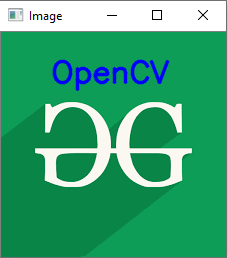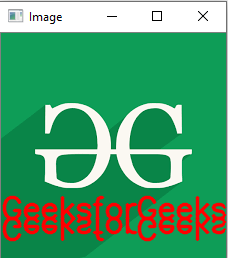# Python OpenCV | cv2.putText() method

OpenCV-Python is a library of Python bindings designed to solve computer vision problems. `cv2.putText()` method is used to draw a text string on any image.

Syntax: cv2.putText(image, text, org, font, fontScale, color[, thickness[, lineType[, bottomLeftOrigin]]])

Parameters:
image: It is the image on which text is to be drawn.
text: Text string to be drawn.
org: It is the coordinates of the bottom-left corner of the text string in the image. The coordinates are represented as tuples of two values i.e. (X coordinate value, Y coordinate value).
font: It denotes the font type. Some of font types are FONT_HERSHEY_SIMPLEX, FONT_HERSHEY_PLAIN, , etc.
fontScale: Font scale factor that is multiplied by the font-specific base size.
color: It is the color of text string to be drawn. For BGR, we pass a tuple. eg: (255, 0, 0) for blue color.
thickness: It is the thickness of the line in px.
lineType: This is an optional parameter.It gives the type of the line to be used.
bottomLeftOrigin: This is an optional parameter. When it is true, the image data origin is at the bottom-left corner. Otherwise, it is at the top-left corner.

Return Value: It returns an image.

Image used for all the below examples:Example #1:

 `# Python program to explain cv2.putText() method ` `   `  `# importing cv2 ` `import` `cv2 ` `   `  `# path ` `path ``=` `r``'C:\Users\Rajnish\Desktop\geeksforgeeks\geeks.png'` `   `  `# Reading an image in default mode ` `image ``=` `cv2.imread(path) ` `   `  `# Window name in which image is displayed ` `window_name ``=` `'Image'` ` `  `# font ` `font ``=` `cv2.FONT_HERSHEY_SIMPLEX ` ` `  `# org ` `org ``=` `(``50``, ``50``) ` ` `  `# fontScale ` `fontScale ``=` `1` `  `  `# Blue color in BGR ` `color ``=` `(``255``, ``0``, ``0``) ` ` `  `# Line thickness of 2 px ` `thickness ``=` `2` `  `  `# Using cv2.putText() method ` `image ``=` `cv2.putText(image, ``'OpenCV'``, org, font,  ` `                   ``fontScale, color, thickness, cv2.LINE_AA) ` `  `  `# Displaying the image ` `cv2.imshow(window_name, image)  `Example #2:

 `# Python program to explain cv2.putText() method ` `   `  `# importing cv2 ` `import` `cv2 ` `   `  `# path ` `path ``=` `r``'C:\Users\Rajnish\Desktop\geeksforgeeks\geeks.png'` `   `  `# Reading an image in default mode ` `image ``=` `cv2.imread(path) ` `   `  `# Window name in which image is displayed ` `window_name ``=` `'Image'` ` `  `# text ` `text ``=` `'GeeksforGeeks'` ` `  `# font ` `font ``=` `cv2.FONT_HERSHEY_SIMPLEX ` ` `  `# org ` `org ``=` `(``00``, ``185``) ` ` `  `# fontScale ` `fontScale ``=` `1` `  `  `# Red color in BGR ` `color ``=` `(``0``, ``0``, ``255``) ` ` `  `# Line thickness of 2 px ` `thickness ``=` `2` `  `  `# Using cv2.putText() method ` `image ``=` `cv2.putText(image, text, org, font, fontScale,  ` `                 ``color, thickness, cv2.LINE_AA, ``False``) ` ` `  `# Using cv2.putText() method ` `image ``=` `cv2.putText(image, text, org, font, fontScale, ` `                  ``color, thickness, cv2.LINE_AA, ``True``)  ` ` `  `# Displaying the image ` `cv2.imshow(window_name, image)  `

Output:Attention geek! Strengthen your foundations with the Python Programming Foundation Course and learn the basics.

To begin with, your interview preparations Enhance your Data Structures concepts with the Python DS Course.

My Personal Notes arrow_drop_upCheck out this Author's contributed articles.

If you like GeeksforGeeks and would like to contribute, you can also write an article using contribute.geeksforgeeks.org or mail your article to contribute@geeksforgeeks.org. See your article appearing on the GeeksforGeeks main page and help other Geeks.

Please Improve this article if you find anything incorrect by clicking on the "Improve Article" button below.

Article Tags :

2

Please write to us at contribute@geeksforgeeks.org to report any issue with the above content.